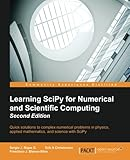# Learning SciPy for Numerical and Scientific Computing, 2nd Edition### Editorial Reviews

Quick solutions to complex numerical problems in physics, applied mathematics, and science with SciPy

• Use different modules and routines from the SciPy library quickly and efficiently
• Create vectors and matrices and learn how to perform standard mathematical operations between them or on the respective array in a functional form
• A step-by-step tutorial that will help users solve research-based problems from various areas of science using Scipy

### Who This Book Is For

This book targets programmers and scientists who have basic Python knowledge and who are keen to perform scientific and numerical computations with SciPy.

### In Detail

SciPy is an open source Python library used to perform scientific computing. The SciPy (Scientific Python) package extends the functionality of NumPy with a substantial collection of useful algorithms.

The book starts with a brief description of the SciPy libraries, followed by a chapter that is a fun and fast-paced primer on array creation, manipulation, and problem-solving. You will also learn how to use SciPy in linear algebra, which includes topics such as computation of eigenvalues and eigenvectors. Furthermore, the book is based on interesting subjects such as definition and manipulation of functions, computation of derivatives, integration, interpolation, and regression. You will also learn how to use SciPy in signal processing and how applications of SciPy can be used to collect, organize, analyze, and interpret data.

By the end of the book, you will have fast, accurate, and easy-to-code solutions for numerical and scientific computing applications.

Chapter 1. Introduction to SciPy
Chapter 2. Working with the NumPy Array As a First Step to SciPy
Chapter 3. SciPy for Linear Algebra
Chapter 4. SciPy for Numerical Analysis
Chapter 5. SciPy for Signal Processing
Chapter 6. SciPy for Data Mining
Chapter 7. SciPy for Computational Geometry
Chapter 8. Interaction with Other Languages

### Book Preview

Click to Look Inside This eBook: Browse Sample Pages